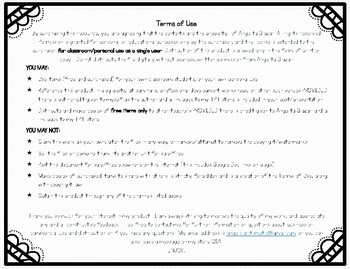# Classifying Numbers - BundleSubject
Resource Type
File Type

Zip

(6 MB)
Product Rating
Standards
• Product Description
• StandardsNEW

This product includes 4 different activities for classifying numbers in the Real Number System:

Classifying REAL Numbers - Includes all Rational and Irrational Numbers

This activity addresses TEKS 8.2A and CCSS.MATH.CONTENT.8.NS.A.1 where students are expected to differentiate between rational and irrational numbers, and use a visual representation to describe relationships between sets of real numbers.

This activity includes:

• Guided notes
• A station activity so students can get additional practice while staying engaged.
• Formative Assessment to check student understanding.

Classifying Rational Numbers - Part 1

This activity addresses TEKS 6.2A where students are required to classify numbers as natural, whole, integer, and rational.

This activity includes:

• Interactive Notebook Notes
• Card sort for differentiating between rational numbers and integers.
• Formative Assessment to check student understanding.

Classifying Rational Numbers - Part 2

This activity addresses TEKS 6.2A where students are required to classify numbers as natural, whole, integer, and rational.

This activity includes:

• A station activity so students can get additional practice while staying engaged.
• A set of task cards to promote higher level thinking.
• Formative Assessment to check student understanding.

Classifying Rational Numbers and Discovering Attributes about each Number Set

The following activity includes 2 different Venn diagrams to be blown up to poster size, and 6 different sets of cards for students to sort into the correct categories.

This activity includes:

• A card sort activity for classifying numbers as Natural, Whole, Integers, or Rational, using a Venn diagram.
• This color coding worksheet can be used as a formative assessment.

----------------------------------------------------------------------------------------------------------------------

You might also like the following 6th grade math resources:

Multiplying Decimals

Properties of Operations

-------------------------------------------------------------------------------------------------------------

Use rational approximations of irrational numbers to compare the size of irrational numbers, locate them approximately on a number line diagram, and estimate the value of expressions (e.g., π²). For example, by truncating the decimal expansion of √2, show that √2 is between 1 and 2, then between 1.4 and 1.5, and explain how to continue on to get better approximations.
Know that numbers that are not rational are called irrational. Understand informally that every number has a decimal expansion; for rational numbers show that the decimal expansion repeats eventually, and convert a decimal expansion which repeats eventually into a rational number.
Understand a rational number as a point on the number line. Extend number line diagrams and coordinate axes familiar from previous grades to represent points on the line and in the plane with negative number coordinates.
Total Pages
N/A
Included
Teaching Duration
N/A
Report this Resource to TpT
Reported resources will be reviewed by our team. Report this resource to let us know if this resource violates TpT’s content guidelines.\$10.25
List Price:
\$13.50
You Save:
\$3.25